This article was published as a part of the Data Science Blogathon.

## Introduction

Exploratory Data Analysis(EDA) is an important component as well as one of the most under-estimated steps in any Data Science project. EDA is essential for well-defined and structured data analysis and should be performed before the machine learning modeling phase.

It involves finding insights from the data upon careful observation and further summarizing its main characteristics. Generally, the real-life data which we work upon contains a lot of ‘noise’, and therefore performing data analysis manually on such datasets becomes a complicated and tedious process.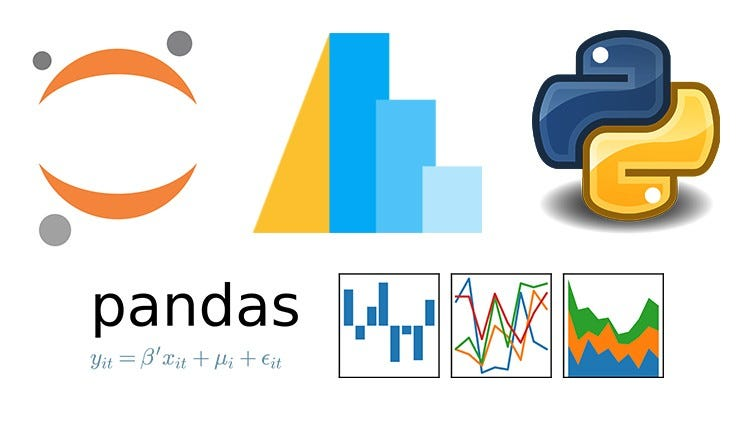Python Getting Started Tutorial: Scientific Calculation with Pandas | by Data Analysis Enthusiast | Medium

Python is one of the most widely used languages for Data Science particularly because of the presence of various libraries and packages that makes data analysis easier.

Accordingly, Pandas is one of the most popular libraries of Python that helps to present the data in a way which is suitable for analysis via its Series and DataFrame data structures. It provides various functions and methods to both simplify as well as expedite the data analysis process.

Here we use  “TITANIC” Dataset to do the practical implementation of all functions.

Firstly, we import Numpy and pandas library and then read the dataset.## Now Let’s Get Started

1. df.head( ): By default, it returns the first 5 rows of the Dataframe. To change the default, you may insert a value between the parenthesis to change the number of rows returned.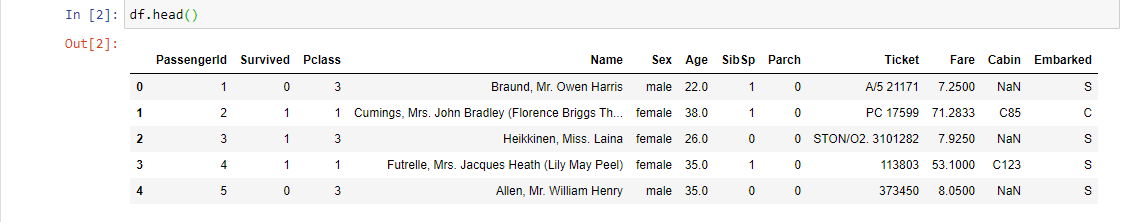2. df.tail( ): By default, it returns the last 5 rows of the Dataframe. This function is used to get the last n rows. This function returns the last n rows from the object based on position.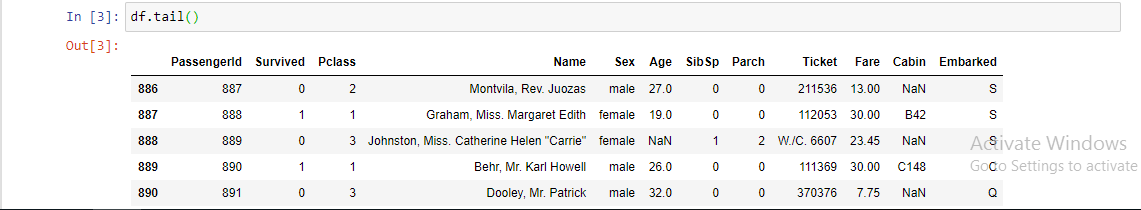3. df.info( ): It helps in getting a quick overview of the dataset. This function is used to get a brief summary of the dataframe. This method prints information about a DataFrame including the index dtype and column dtypes, non-null values, and memory usage.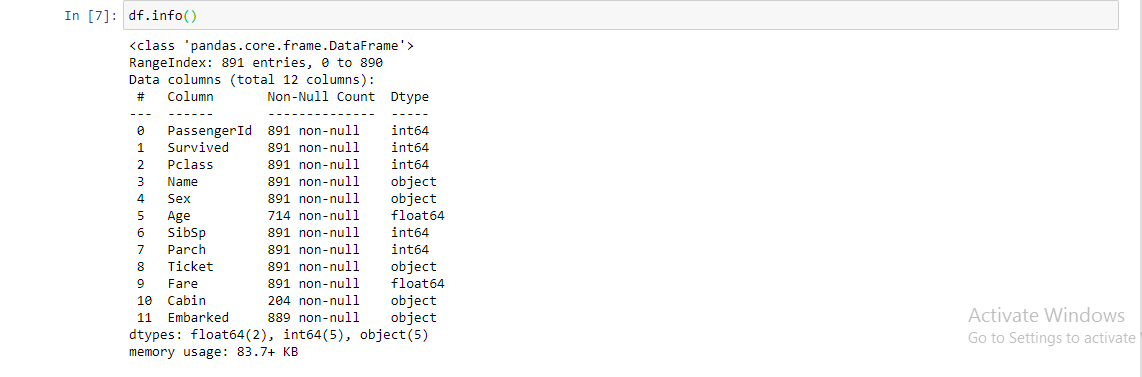4. df.shape: It shows the number of dimensions as well as the size in each dimension. Since data frames are two-dimensional, what shape returns is the number of rows and columns.5. df.size: Return an int representing the number of elements in this object. Return the number of rows if Series, otherwise returns the number of rows times the number of columns if DataFrame.6. df.ndim: Returns dimension of dataframe/series. 1 for one dimension (series), 2 for two dimensions (dataframe).7. df.describe( ): Return a statistical summary for numerical columns present in the dataset. This method calculates some statistical measures like percentile, mean and standard deviation of the numerical values of the Series or DataFrame.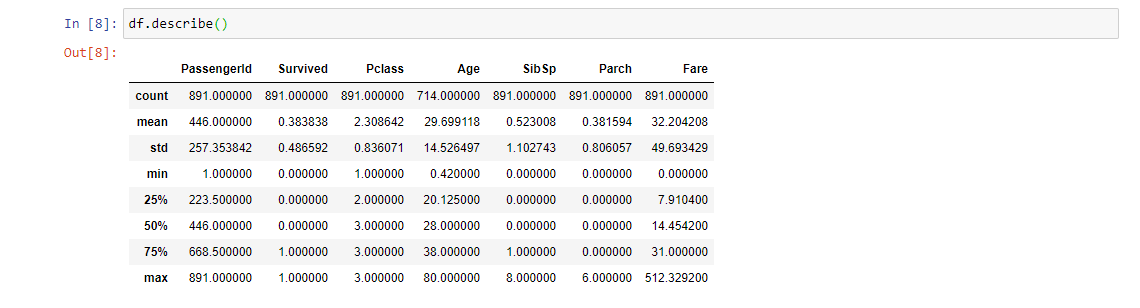8. df.sample( ): Used to generate a sample randomly either row or column. It allows you to select values randomly from a Series or DataFrame. It is useful when we want to select a random sample from a distribution.9. df.isnull( ).sum( ): Return the number of missing values in each column.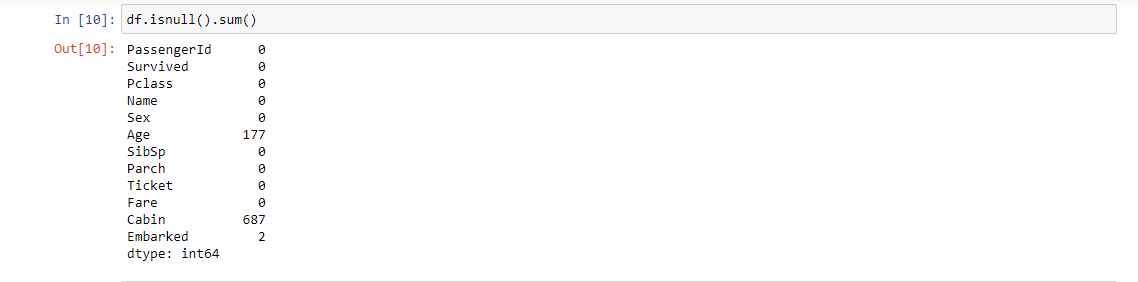10. df.nunique( ): Return number of unique elements in the object. It counts the number of unique entries over columns or rows. It is very useful in categorical features especially in cases where we do not know the number of categories beforehand.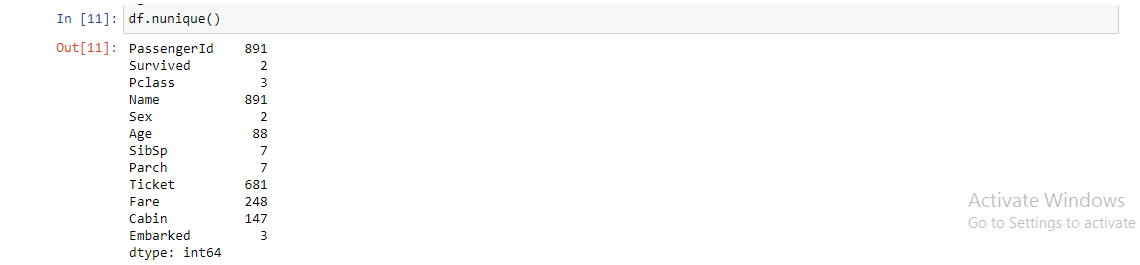11. df.index: This function searches for a given element from the start of the list and returns the lowest index where the element appears.12. df.columns: Return the column labels of the dataframe.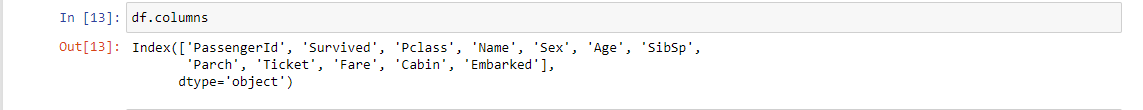13. df.memory_usage( ): Returns how much memory each column uses in bytes. It is useful especially when we work with large data frames.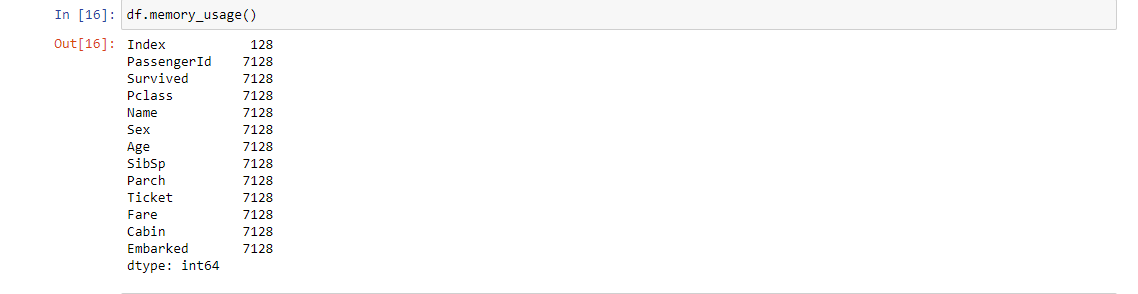14. df.dropna( ): This function is used to remove a row or a column from a dataframe that has a NaN or missing values in it.15. df.nlargest( ): Returns the first n rows ordered by columns in descending order.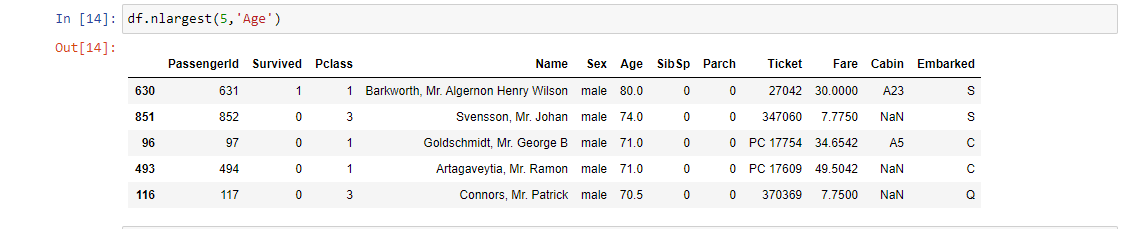16. df.isna( ): This function returns a dataframe filled with boolean values with true indicating missing values.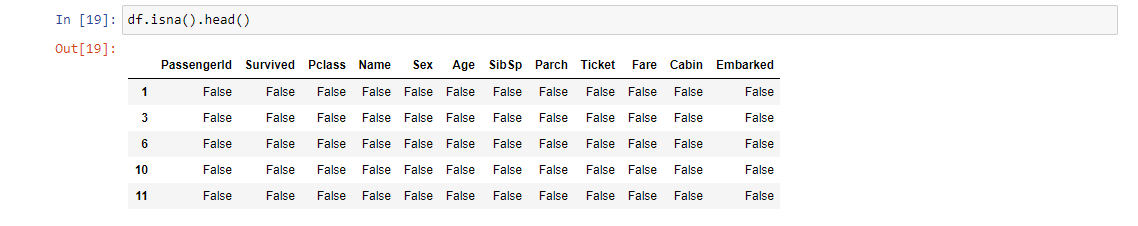17. df.duplicated( ):  Returns a boolean Series denoting duplicate rows.18. value_counts( ): This function is used to get a Series containing counts of unique values. The resulting object will be in descending order so that the first element is the most frequently occurring element. It excludes missing values by default. This function comes in handy when we want to check the problem of class imbalance for a categorical variable.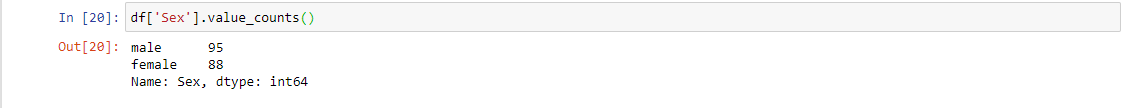19. df.corr( ): This function is used to find the pairwise correlation of all columns in the dataframe. Any missing values are automatically excluded. For any non-numeric data type columns in the dataframe, it is ignored. This function comes in handy while we doing the Feature Selection by observing the correlation between features and target variable or between variables.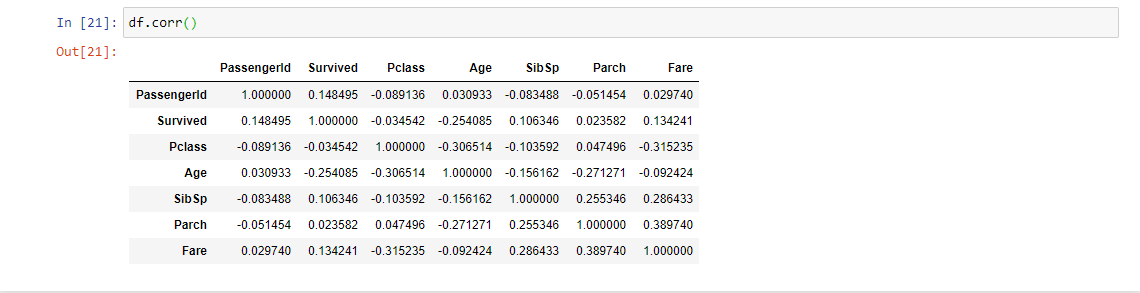20. df.dtypes: This function shows the data type of each column.## End Notes

If you liked this and want to know more, go visit my other articles on Data Science and Machine Learning by clicking on the Link

Something not mentioned or want to share your thoughts? Feel free to comment below And I’ll get back to you.

Till then Stay Home, Stay Safe to prevent the spread of COVID-19, and Keep Learning!

## Chirag Goyal

Currently, I pursuing my Bachelor of Technology (B.Tech) in Computer Science and Engineering from the Indian Institute of Technology Jodhpur(IITJ). I am very enthusiastic about Machine learning, Deep Learning, and Artificial Intelligence.

The media shown in this article are not owned by Analytics Vidhya and is used at the Author’s discretion.